Next: Flux Freezing Up: Magnetohydrodynamic Fluids Previous: Introduction

# Magnetic Pressure

The MHD equations can be combined with Maxwell's equations,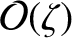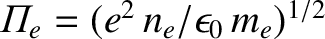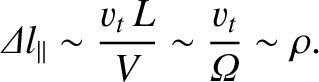(687)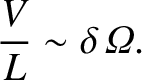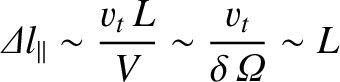(688)

to form a closed set. The displacement current is neglected in Eq. (687) on the reasonable assumption that MHD motions are slow compared to the velocity of light. Note that Eq. (688) guarantees that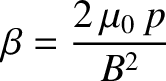, provided that this relation is presumed to hold initially. Similarly, the assumption of quasi-neutrality renders the Poisson-Maxwell equation,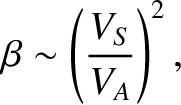, irrelevant.

Equations (682) and (687) can be combined to give the MHD equation of motion: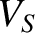(689)

where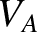(690)

Suppose that the magnetic field is approximately uniform, and directed along the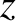-axis. In this case, the above equation of motion reduces to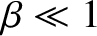(691)

where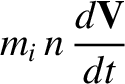(692)

Note that the magnetic field increases the plasma pressure, by an amount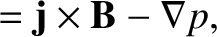, in directions perpendicular to the magnetic field, and decreases the plasma pressure, by the same amount, in the parallel direction. Thus, the magnetic field gives rise to a magnetic pressure,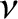, acting perpendicular to field-lines, and a magnetic tension,, acting along field-lines. Since, as we shall see presently, the plasma is tied to magnetic field-lines, it follows that magnetic field-lines embedded in an MHD plasma act rather like mutually repulsive elastic bands.Next: Flux Freezing Up: Magnetohydrodynamic Fluids Previous: Introduction
Richard Fitzpatrick 2011-03-31